Artificial intelligence seems to be everywhere these days. The news has stories about poetry-writing AI, the experts consider AI “the new electricity” and even AI whiskey is going to make an appearance soon. When you try reading these articles, there’s usually a flood of information coming at you. Words like models, neural networks, and deep learning crop up frequently. But what do these concepts even mean? With this series of blog posts, we’re going to address all the questions you’ve ever had about this topic and take you on an amazing adventure.

In this first part, we’re going to introduce the concepts of machine learning, neural networks, and deep learning. You don’t need any previous knowledge about these topics to follow this article, so settle in and keep reading!

Making Your Knowledge Functional

Let's start with a basic concept: functions. Yes, like the ones that you learned about in your math class. They take a number, perform some calculations with it, and produce another number.

y=f(x)
Given f and x, we calculate y.

You can describe all computer software as functions of some kind. They take an input value—something the user enters—and provide some output. This output is then either displayed on the screen, stored in a file, or sent to the internet.  You've probably heard that programmers write software. What it usually means is that they're writing the function f that acts on the inputs the user enters.

So What Is Machine Learning Anyway?

In machine learning, the programmer doesn't write the function f for the computer to apply it. Instead, it's up to the computer to learn the function f.

(X,Y)→f
Given X and Y, we learn a function f that turns X into Y.

This process is much harder for a computer. But it can be quite useful, especially when we know how to turn X into Y, but we don't know how to describe it as a function.

Consider the task of distinguishing between pictures of cats and dogs. It's so easy that any four-year-old can do it. But it's difficult to describe how to make the distinction. Both kinds of animals have eyes, ears, fur, and tails. So how can you teach someone to distinguish between cats and dogs?

You show them some pictures of both animals and soon they can differentiate between a cat and a dog. We can also describe this as providing X (pictures) and Y (respective classification) to let a person learn f.

A lot of tasks fall into this category. They're often difficult to describe, but we can do them based on our experience and intuition. In tasks like these, machine learning thrives. Some examples are image classification, translation, summarization, fraud detection and so on.

Because the goal for the function is provided, this kind of machine learning is called supervised learning. In contrast, when only the inputs (X) are provided and the task is to find structure within those inputs, it's called unsupervised learning. You can use groups or clusters with some commonality for unsupervised learning.

Let’s Start Learning Functions

So now that we've established that learning functions is useful, let's look into how we can do so. How can I learn a function that distinguishes between the pictures of cats and dogs? Or one that predicts tomorrow's lottery winning entry? Those are tricky problems. We can start by narrowing the scope of functions we're learning to a subset of functions of a more basic form—linear functions.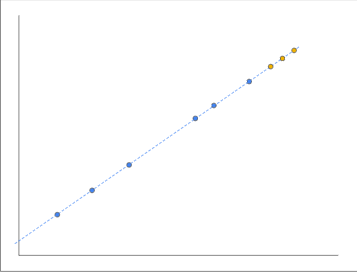f(x)=wx+b

You can always reduce a linear function to the following basic structure:

Take an input x, multiply it by a weight w and add a bias b.

Here, learning the function f is figuring out the adequate values for w and b. It's like solving equations. If that isn't one of your strengths, worry not, because that isn't the solution, either. Solving equations works great when you have exact solutions. In most real-world data, you only have approximate solutions. As a result, you don't try to find the solution in most machine learning problems. Instead, you try to find the best fit.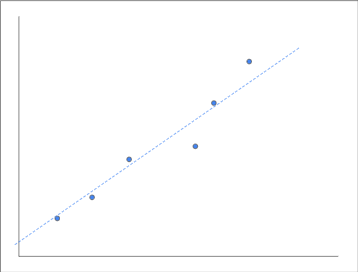While we can find trends in most real-world data, it is unusual to have the data exactly on the trend line.

Learning our linear function is thus like learning w and b that provide the best fit for the data we have. We can iterate that with small changes to w and b that adjust our function closer to the goal.

Machine learning frameworks such as Tensorflow help you with learning these linear functions. You can provide the type of function you're learning (in this case, linear), some data, and a cost function. The cost function shows how far the data predicted by f is from the goal. You can adjust the variables (like w and b) towards minimizing the cost function. Minimizing the cost function lets the predicted data be closer to the goal.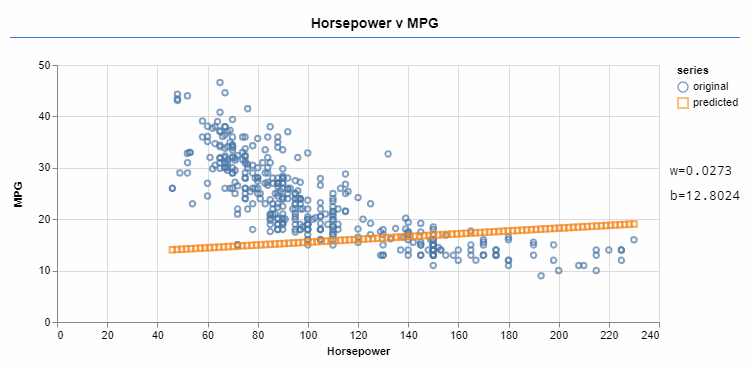An optimizer iteratively changes the values of w and b, approximating the function to the target data.

The Lack of Linearity in the World

However, all functions aren't linear. A lot of the real-world problems to be solved aren't linear either. Fitting a linear function to a non-linear problem can result in a poor solution.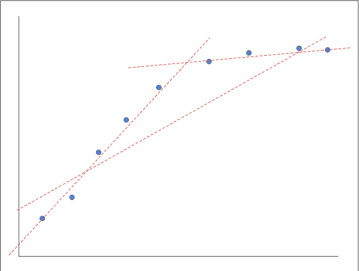Consider this example. This nonlinear problem can be well fit by two distinct linear functions over different segments. However, you cannot fit it well using a single linear function. We already know that we can train two linear functions f1 and f2, one for each segment. But can they be combined so that one of the functions is active in only a given segment?

Let us take a look at the standard logistic sigmoid function. It's a nonlinear function with an interesting shape that goes smoothly from 0 to 1.

This is interesting because by multiplying it with a value we can:

• Mute that value (if we're on the 0-side)
• Leave it be (if we're on the 1-side)
• Do some cross-fade (if we're in the middle).

Thus we see that this function almost acts like a switch.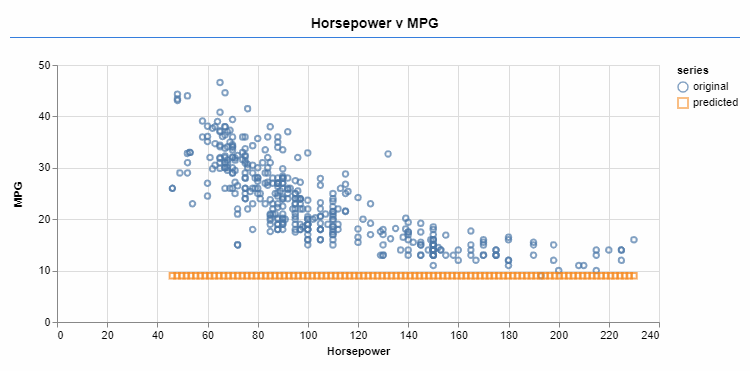An optimizer iteratively changes the function parameters. The sigmoid activation allows a better fit for the target data.

Stacking some linear functions results in yet another linear function. The answer lies in stacking pairs of linear functions with non-linear activations (such as the sigmoid function). This results in a combined non-linear function that is increasingly more expressive.

It’s Getting Colder, Layer Up With Deep Learning

The stacked pairs of linear functions with non-linear activations are called layers. The more layers a network has, the more difficult it will be to train it. But it will also become more powerful when it comes to the ability to fit your data. If we visualize these many layers, the network appears to have depth. As a result, using many layers in a network is called deep learning.

This brings us to another related term we hear quite a lot—a model.  A model is the learned function that we've been referring to as f so far. Recently, adding more layers has been part of the strategy to get more powerful machine learning models.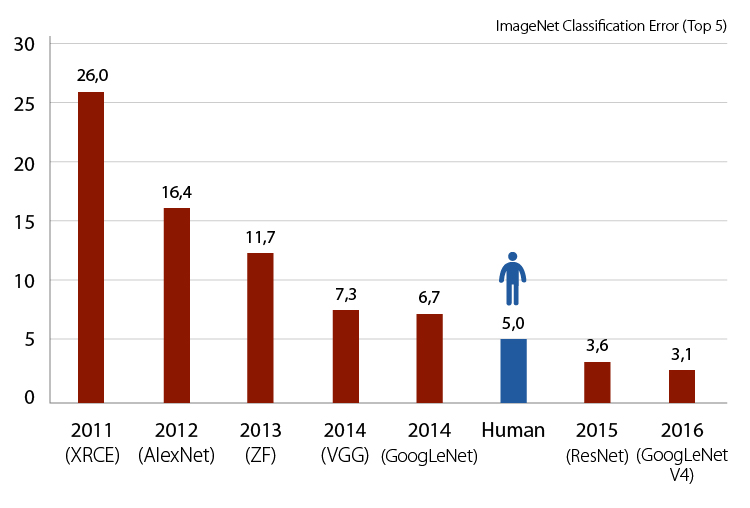For ImageNet, a well-known image classification benchmark, smaller error rates (up to superhuman performance) have been achieved by deeper networks.

Numbers Here, Numbers There, Numbers Everywhere

We've simplified the problem of learning arbitrary functions. We now know that it is similar to adjusting numbers in functions that take numbers and output numbers. So, how do we go from numbers to pictures and categories?

For computers, a picture is essentially numbers. Pictures are represented in pixels, which have color intensities. So we can decompose any picture to a matrix of numbers that correspond to those intensities.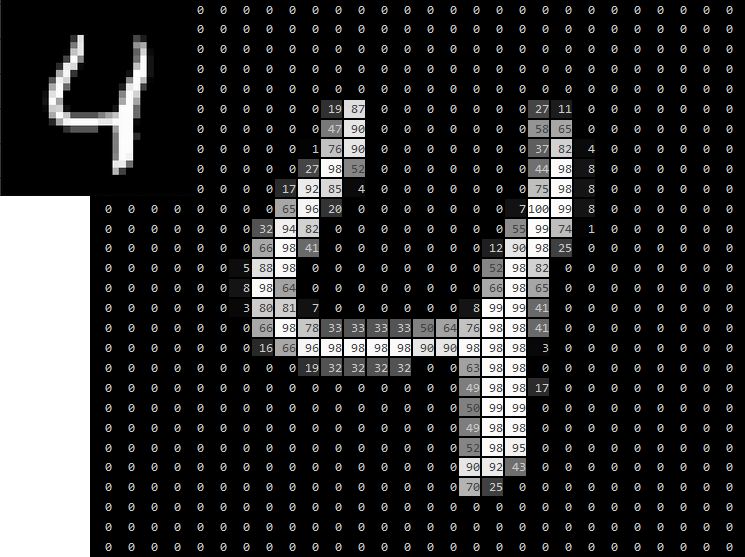If we get a matrix of weights with the right shape and do matrix multiplication, we can go from pixel intensities to category scores. For a task such as recognizing handwritten digits, we need ten categories (one for each digit). The weights in the weight matrix must be learned in a way such that higher pixel intensities result in higher scores in a given area and vice-versa.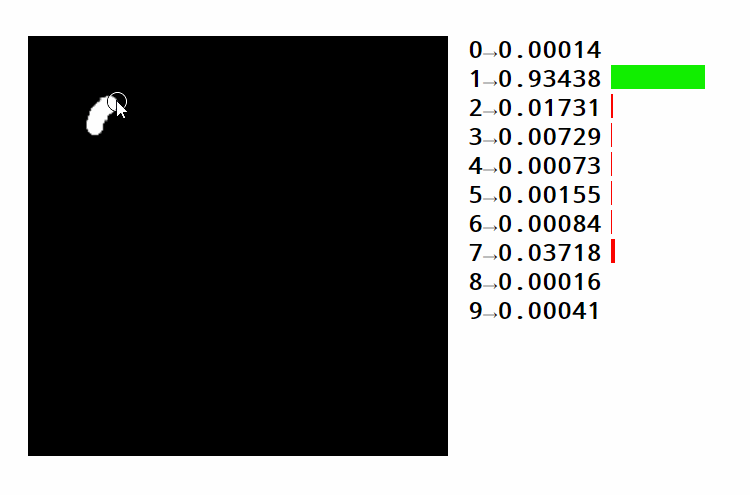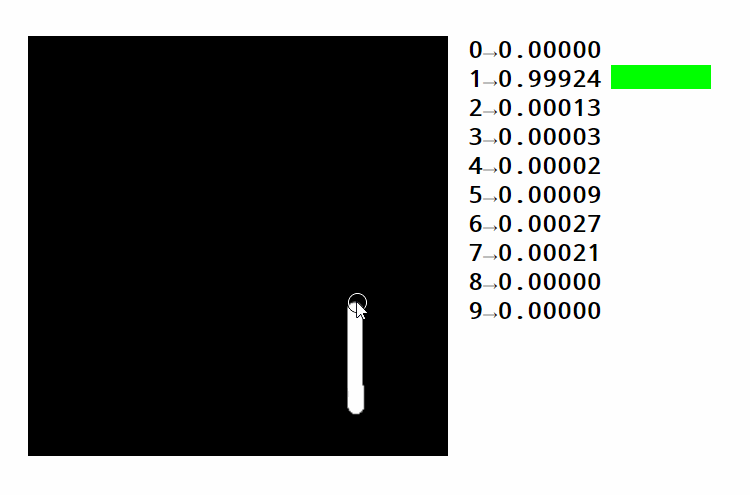Take a look at this example. You can see how different areas activate different digits. You can also see how the same strategy for finding numbers can be used to classify images. In this case, we're distinguishing pictures of handwritten digits over 10 categories. Text is usually encoded by using a sequence of numbers. These numbers either represent the position of each word in a dictionary or the position of each character in the alphabet. Both approaches have advantages and disadvantages that we're not getting into now.

Here's what we've talked about so far:

• Finding functions (similar to finding numbers)
• Processing images and text (similar to processing numbers)
• Categorizing or picking the result slot with the highest number

Structure of Neural Networks: It’s a Convoluted Matter

Let's go back to our image recognition example. The areas marked green in the following image would likely activate the category for the number four. As the space at the top reduces, turning the four into a nine, the score for four keeps getting lower.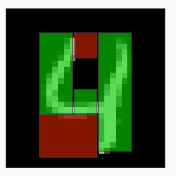However, it's easy to see how even slight changes in the input could make it fail to match the appropriate areas. This would cause the network to provide the wrong results.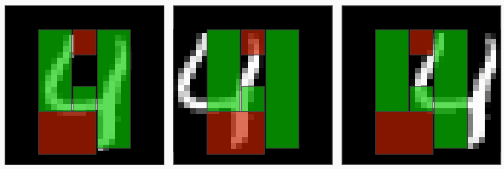So what is the problem over here? What makes a number look like four is not the fact that a particular area of an image has ink or no ink. It's how the inked lines are matched against each other, independent of their absolute placement in the image. With a single matrix multiplication, we can't have a notion of independent lines and shapes of the image. We can only score categories based on absolute positions of the image.

Let's think of a different mechanism over here. The system could first identify lines and intersections in the image. Then, it could feed that information to a second system. This system would then have an easier time scoring categories based on how the lines and intersections were made.

We've already made matrix multiplication over a full image yield a digit category. Now we could make a smaller matrix multiplication over a segment of the image yield basic information about that segment. Instead of scoring a digit category, we'd be scoring categories for lines, intersections, or emptiness.

Let's say we perform the same multiplication over several tiled segments. We could then obtain a set of tiled outputs that would have a spatial relation with the original image. These tiles would have some richer shape information instead of pixel intensities. This repetition of the same operation over different segments with tiling the results is called a convolution. Neural networks that use this strategy are called Convolutional Neural Networks (CNNs).

Under convolutions, the same operation is independently applied for many image segments. Like the layers before, we can also stack convolution layers. The outputs for each segment would then be the input for the next layer. Irrespective of its absolute position in the canvas, a convolution can recognize information. This is because of the tiling. However, as it looks at a segment (rather than a single point) and because the tiles can overlap, stacked convolutions can make use of surrounding information. This makes them a good fit for image processing.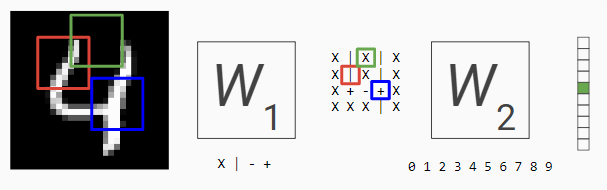For instance, let’s consider the image above. The original image has 28x28 elements with one dimension of intensity. It is converted into an image of 4x4 with four dimensions of intensity. It is then converted into a single element with 10 dimensions of intensity, representing the ten categories we’re classifying over.

In traditional convolutions, the input starts with a high number of elements (pixels). The amount of information per element is kept low (just the color intensity). As we go through the layers, the number of elements decrease while the quantity of information per element increases. Color intensities are processed into category scores. They are then further processed into more categories.

Let's think of object detection in a complex scene.

• The first layers would learn how combining different pixel intensities represent basic shape categories (such as line and corner).
• The next layers would learn how combinations of such basic shapes could yield more complex shapes (such as door or eye).
• The final layers would learn how the combination of those could produce even more complex categories (such as a face or house).

CNN-based architectures are well suited for image processing problems. All state-of-the-art image models now have CNNs in their core. This is because of how the architecture is close to the problem at hand. When processing images, we're concerned with shape compositions made of simpler shapes.

If you’d like to learn more about CNNs, take a look at this excellent chapter on Convolutional Neural Networks from Stanford’s CS231n notes.

A Recurring Subject

We read text using our eyes and interpret each word with our brains. It's a complicated process. We manage to keep tabs on the words we already read. These words then form a context. This context is further enriched with each new word we read, until it forms the entire sentence.

A neural network that processes sequence can follow a similar scheme. The processing unit starts with an empty context. By taking each sequence element as an input, it produces a new version of the context.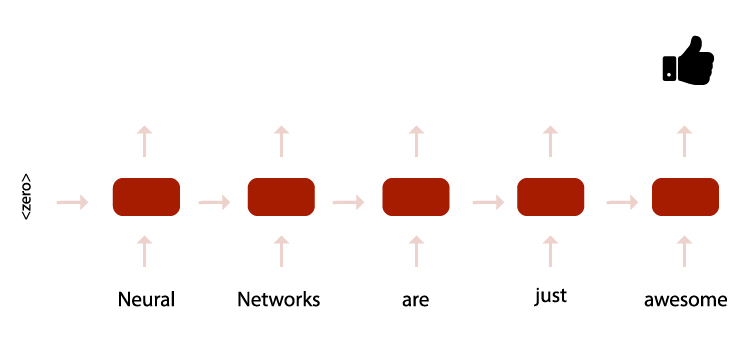Classifying sentiment on a sentence with a NN — each block processes a word and passes context to the next until a classification is achieved at the end.

This processing unit takes as input a previous output of itself. Thus it's called a recurrent unit.

Recurrent networks are harder to train. By feeding the outputs as inputs to the same layer, we create a feedback loop that can cause small perturbations. These small disturbances are then recurrently amplified in the loop, causing big changes in the final result.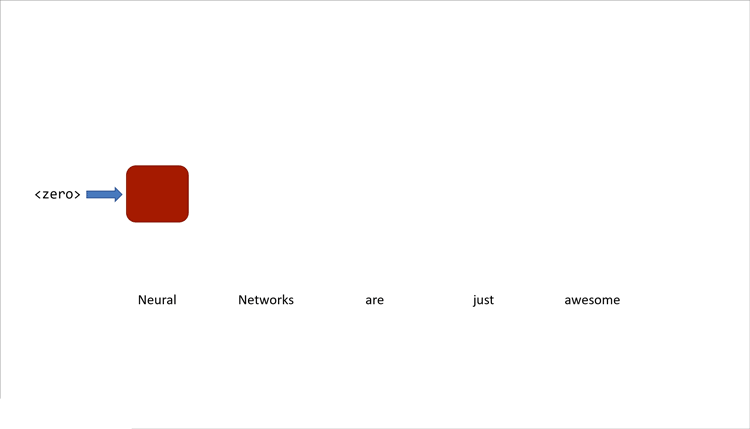In an RNN, the same block is reused for all items in the sequence. The context of a previous timestep is passed on to the next timestep.

This instability is a price to pay for recurrence. It is compensated by the fact that these networks are great at tasks of sequence processing. Recurrent Neural Networks (RNNs) are used in most state-of-the-art models for text and speech processing. They're used for tasks like summarization, translation or emotion recognition.

If you’d like to know more about how RNNs work and how well they perform both in classifying and in generating text, we recommend this cool blog post by Andrej Karpathy on the unreasonable effectiveness of Recurrent Neural Networks.

To be Architected...

So far, we have talked about dense neural networks. Here everything in a layer connects with everything in the previous layer. These networks are of the simplest kind. We've spoken of CNNs and how they are good for image processing. We discussed RNNs and how they're good for sequence processing.

In today’s world, neural architecture matters a lot. There are a lot of variations and combinations of these kinds of architectures. For instance, processing video, which can be thought of as a sequence of images seems to be a task for a RNN. These RNNs, in turn, have recurrent units that use a CNN. There's also a growing field of research on automatically tailoring neural network architectures for particular types of tasks.

But there are types of data for which these architectures are unfit. One example would be graphs. A new type of neural network architectures called Graph Neural Networks have been emerging recently. In the next part of this series, we'll focus on these GNNs. Check it out